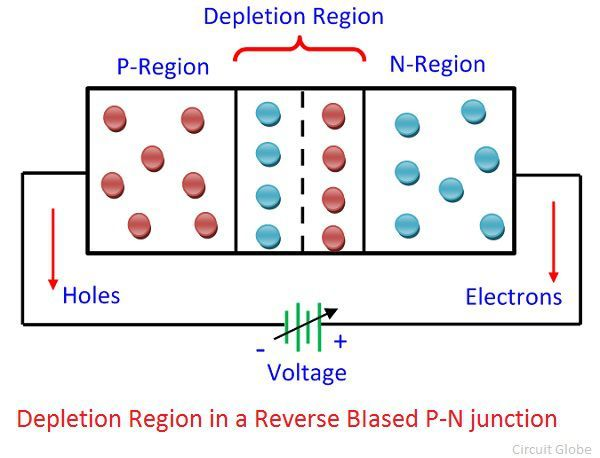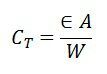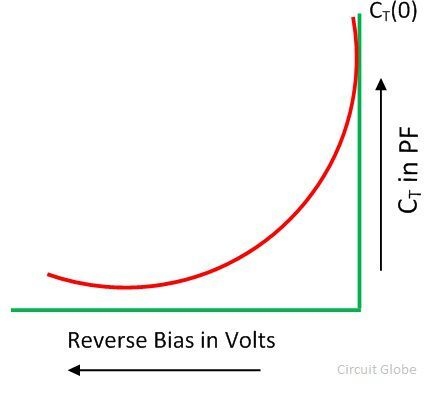# Varactor Diode

Definition: The diode whose internal capacitance varies with the variation of the reverse voltage such type of diode is known as the Varactor diode. It is used for storing the charge. The varactor diode always works in reverse bias, and it is a voltage-dependent semiconductor device

The voltage-dependent device means the output of the diode depends on their input voltage. The varactor diode is used in a place where the variable capacitance is required, and that capacitance is controlled with the help of the voltage. The Varactor diode is also known as the Varicap, Voltcap, Voltage variable capacitance or Tunning diode.

## Symbol of Varactor Diode

The symbol of the varactor diode is similar to that of the PN-junction diode. The diode has two terminals namely anode and cathode. The one end of a symbol consists the diode, and their other end has two parallel lines that represent the conductive plates of the capacitor. The gap between the plates shows their dielectric.## Working of Varactor Diode

The Varactor diode is made up of n-type and p-type semiconductor material. In an n-type semiconductor material, the electrons are the majority charge carrier and in the p-type material, the holes are the majority carriers. When the p-type and n-type semiconductor material are joined together, the p-n junction is formed, and the depletion region is created at the PN-junction. The positive and negative ions make the depletion region. This region blocks the current to enter from the PN-region.The varactor diode operates only in reverse bias. Because of reverse bias, the current does not flow. If the diode is connected in forward biasing the current starts flowing through the diode and their depletion region become decreases. The depletion region does not allow the ions to move from one place to another.

The Varactor diode is used for storing the charge not for flowing the charge. In the forward bias, the total charge stored in the diode becomes zero, which is undesirable. Thus, the Varactor diode always operates in the reverse bias.

The formula gives the capacitance of varactor diode,Where, ε – Permittivity of the semiconductor material.
A – area of PN-junction
W – width of depletion region

The capacitance of the varactor diode increases with the increase of n and the p-type region and decreases with the increases of the depletion region. The increase in capacitance means the more charges are stored in the diode. For increasing the storage capacity of charge the depletion region (which acts as a dielectric of the capacitor) of the diode should be kept small.

### Characteristic of Varactor Diode

The characteristic curve of the varactor diode is shown in the figure below.  The graph shows that when the reverse bias voltage increases the depletion region increases, and the capacitance of the diode reduces.The following are the advantages of the varactor diode.

1. The varactor diode produces less noise as less compared to the other diode.
2. It is less costly and more reliable.
3. The varactor diode is small in size and less in weight.

### Varactor diode in tunning Circuit

The figure below shows that D1 and D2 are the two Varactor diode. These diodes provide the variable resistance in the parallel resonance circuit. The Vc is the DC voltage used for controlling the reverse voltage of the diode.The L is the inductance of the circuit, and it is measured in Henry. The resonant frequency of the circuit is expressed as C1 and C2 is the maximum voltage capacitance of the diode